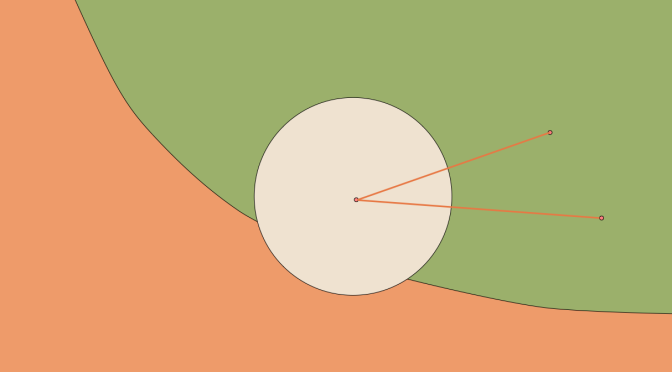# An unbounded convex not containing a ray

We consider a normed vector space $$E$$ over the field of the reals $$\mathbb{R}$$ and a convex subset $$C \subset E$$.

We suppose that $$0 \in C$$ and that $$C$$ is unbounded, i.e. there exists points in $$C$$ at distance as big as we wish from $$0$$.

The following question arises: “does $$C$$ contains a ray?”. It turns out that the answer depends on the dimension of the space $$E$$. If $$E$$ is of finite dimension, then $$C$$ always contains a ray, while if $$E$$ is of infinite dimension $$C$$ may not contain a ray.

## Finite-dimensional case

Even if it means reducing the dimension of the space, we can suppose that $$0$$ is an interior point of $$C$$. By hypothesis, there exists a sequence $$(y_n)_{n \in \mathbb{N}}$$ with $$y_n \in C$$ and $$\Vert y_n \Vert > n$$ for all $$n \in \mathbb{N}$$. The sequence $$(\frac{y_n}{\Vert y_n \Vert})_{n \in \mathbb{N}}$$ belongs to the unit sphere which is compact as $$E$$ is supposed finite-dimensional. Therefore it has a converging subsequence (this is the Bolzano-Weierstrass theorem). By renumbering the sequence $$(y_n)_{n \in \mathbb{N}}$$, we can even suppose that $$(\frac{y_n}{\Vert y_n \Vert})_{n \in \mathbb{N}}$$ itself converge to a vector $$v$$.

Let’s prove that $$C$$ contains points as far as we want from $$0$$ on the line $$\mathbb{R} v$$. We pick-up a real $$A > 0$$. There exists $$N \in \mathbb{N}$$ such that $$\Vert y_n \Vert \ge A$$ for all $$n \ge N$$. As $$0 \in C$$ and $$C$$ is supposed convex, the points $$w_n = A \frac{y_n}{\Vert y_n \Vert}$$ belongs to $$C$$ for $$n \ge N$$. Moreover the sequence $$(w_n)_{n \in \mathbb{N}}$$ converges to $$A v$$. As $$0$$ is supposed to be an interior point of $$C$$, $$w_n – Av \in C$$ for $$n$$ big enough. Then also:
$\frac{1}{2}A v= \frac{1}{2}(w_n+(A v -w_n)) \in C$ Finally, the ray $$[0,+\infty v)$$ belongs to $$C$$.

## A counter-example in the infinite-dimensional case

Let’s take a sequence $$\textbf{e}=(e_n)_{n \in \mathbb{N}}$$ of linearly independent vectors whose sequence of norms converges to $$+ \infty$$ and for $$C$$ the convex hull of that sequence. If $$C$$ contains a ray, it also contains two points $$a$$ and $$b$$ that are convex combinations of vectors of the sequence $$\textbf{e}$$. Therefore, $$a$$ and $$b$$ belongs to a finite-dimensional space $$F=\mathbb{R}e_1 + \cdots + \mathbb{R}e_N$$ as well as the ray that is supposed to be contained in $$C$$. But $$C \cap F$$, which is the convex hull of $$\{e_1, \dots, e_n\}$$ is compact (see here), a contradiction.